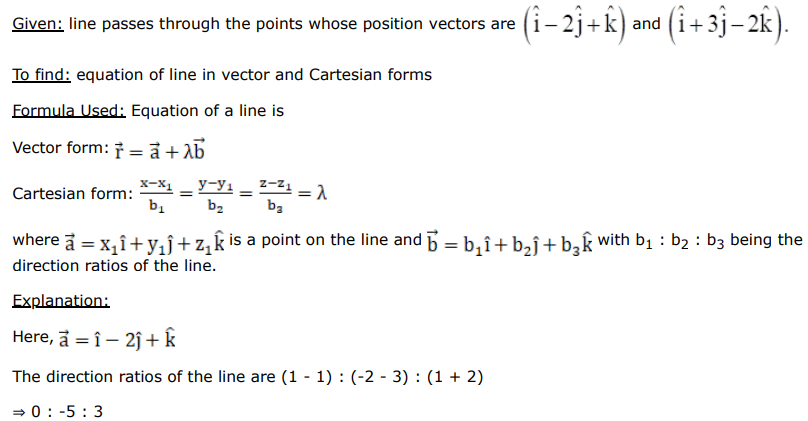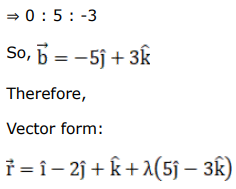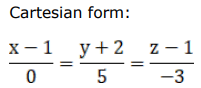# Find the vector and Cartesian equations of the line joining the points whose position vectors areQuestion:

Find the vector and Cartesian equations of the line joining the points whose position vectors are $(\hat{\mathrm{i}}-2 \hat{\mathrm{j}}+\hat{\mathrm{k}})$ and $(\hat{\mathrm{i}}+3 \hat{\mathrm{j}}-2 \hat{\mathrm{k}})$

Solution: